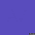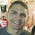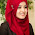# Difference between == and === Equal Operator in JavaScript

In one of the recent JavaScript interview for a Java web development position, one of my readers was asked this questions, What is the difference between comparing variables in JavaScript using "==" and "===" operator?  My reader got shocked because he was from Java background and doesn't have great exposure to JavaScript, though he was pretty much familiar with some JavaScript function, event handling, and some jQuery tricks, he wasn't aware of subtle details of JavaScript. He did the right think, politely said that he is not aware of the difference between == and === operator. Though It did not affect his interview performance much, he was keen to know about this as soon as he finished his interview. He asked to me as well, and that's the reason of this post. In one word, main difference between "==" and "===" operator is that formerly compares variable by making type correction e.g. if you compare a number with a string with numeric literal, == allows that, but === doesn't allow that, because it not only checks the value but also type of two variable, if two variables are not of the same type "===" return false, while "==" return true.

We will see couple of example of both operator in this article, to understand difference between them much better.

## Difference between == and ===  in JavaScript

Since JavaScript support both strict equality and type-converting equality, it's important to know which operator is used for which operation. As I said that, === takes type of variable in consideration, while == make type correction based upon values of variables, following are couple of more differences between "==" and "===" operator in JavaScript programming language :

1) When we compare two variables of different type e.g. a boolean with a string or a number with String using == operator, it automatically converts one type into another and return value based upon content equality, while === operator is strict equality operator in Java, and only return true if both variable of same type and also contains same value. This will be much clear with following example of == and === operator in JavaScript :

```0==false   // true, because false is equivalent of 0
0===false  // false, because both operands are of different type
2=="2"     // true, auto type coercion, string converted into number
2==="2"    // false, since both operands are not of same type```

2)  "==" operator is known as type coercion operator and anytime if both values are same and compared using ==operator, type coercion happens. On the other hand === is known as strictly equality operator. It's much similar Java's equality operator (==), which gives compilation error if you compare two variables, whose types are not compatible to each other. In fact, you should always use "===" operator for comparing variables or just for any comparison.

3)  While comparing variable using strict equality operator in Java, two object are strictly equal to each other if both are of same type and they refer to same instance. Similarly two String are equal to each other if contents of each others are same e.g. same characters at same position. Another good follow-up question is what would be result of comparing null and undefined using == and === operator in JavaScript. Well answer is simple, when you use "==" operator it will return true, while if you use "===" or strict equality operator, it will return false.

4) One more follow-up question of == vs === operator is difference between != and !== operator in JavaScript. I think it's pretty easy to deduct that !== operator is strict non equality operator, which will take type into consideration while comparing two variables or two values in JavaScript.

5) Last but not the least question related to this JavaScript interview question is, what happen if you compare Nan with any number or Nan itself, using  === operator,  You should remember that NaN (Not a Number) is not equal to anything, including NaN.

That's all about difference between "==" and "===" JavaScript operator, you should always use strict equality operator i.e. === and avoid using == operator even if you need type coercion, instead convert the type by yourself to make it more readable. By the way, it's one of the interesting JavaScript interview Question, and you can expect in beginner level JavaScript interviews, much to the same, where Java developers are expected to know about client side tools e.g. HTML, CSS and JavaScript.

Further Learning
JavaScript Fundamentals by Liam McLennan
ES6 Javascript: The Complete Developer's Guide
JavaScript: Understanding the Weird Parts

Related Programming articles from Java67 Blog

10 Frequently asked SQL Query Interview Questions
Difference between wait and sleep in Java
Difference between primary and unique key in SQL
Difference between clustered and non-clustered index in SQL

1.Though many people suggest that use triple plus operator, almost all Javascript code I see, they end up using double plus (==) operator.

2.Thanks lot .. its very useful

3.4.5.Thank you for the info!!

6.7.Thank you, good explanation and clear example

8.Typo: replace Java to JavaScript in line "equality, while === operator is strict equality operator in Java, and only return true if both variable of ".

9.Thanks a lot for this info

10.Great article, I too didn't know the difference between == and ===.

Just curious, how would you tell if something equals NaN? As you mentioned, NaN !== NaN.